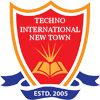# Power Electronics Lab

PC-EE 593 (5th Semester)

Major Equipments and Software:

• Hardware Kit for synchro Transmitter & Receiver with position Control.
• Process control Simulator (for linear system & PID Study).
• Dual Trace Digital storage Oscilloscope.
• DC Motor Speed and Position Control system with
• Analog PID controller
• Digital controller based on Arduino and computer Interfacing.
• MATLAB

Experiment List:

• Familiarization with MAT-Lab control system toolbox, MAT-Lab- Simulink toolbox
• Determination of Step response for first order & Second order system with unity feedback with the help of CRO & calculation of control system specification, Time constant, % peak overshoot, settling time etc. from the response.
• Simulation of Step response & Impulse response for type-0, type-1 & Type-2 system with unity feedback using MATLAB
• Determination of Root locus, Bodeplot, Nyquist plot using MATLAB control system toolbox for a given system & stability by determining control system specification from the plot.
• Determination of PI, PD and PID controller action of first order simulated process.
• Determination of approximate transfer functions experimentally from Bode plot.
• Evaluation of steady state error, setting time, percentage peak overshoot, gain margin, phase margin with addition of Lead, Lag, Lead-lag compensator.
• Study of a practical position control system obtaining closed step responses for gain setting corresponding to overdamped and under-damped responses. Determination of rise time and peak time using individualized components by simulation. Determination of un-damped natural frequency and damping ratio from experimental data.
• Analysis of performance of Lead, Lag and Lead-Lag compensation circuits for a given system using simulation.
• Determination of Transfer Function of a given system from State Variable model and vice versa.
• Analysis of performance of a physical system using State variable technique by simulation. Study of step response and initial condition response for a single input, two-output system in SV form by simulation.

Beyond the Curriculum:

• Demonstration of real-time position control system with PC based motor control control setup.
• Demonstration of tuning of P, PI and PID controller for PC based position control system.